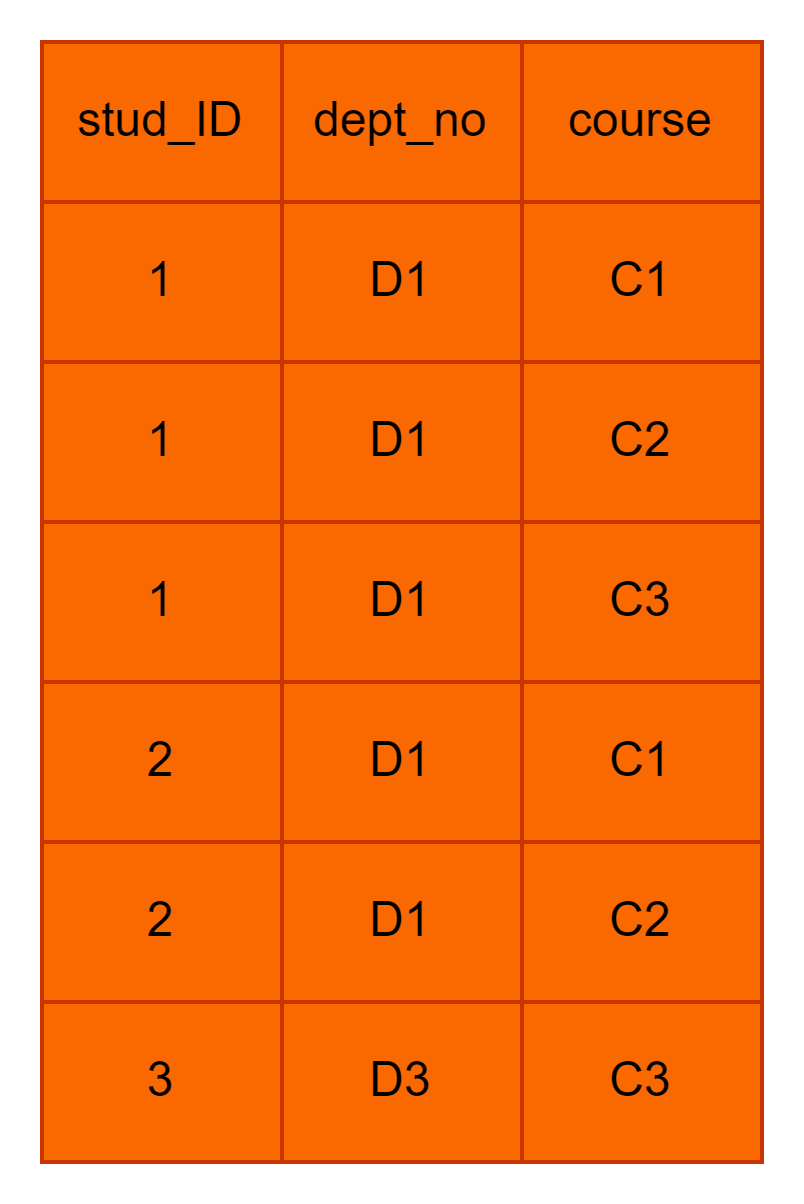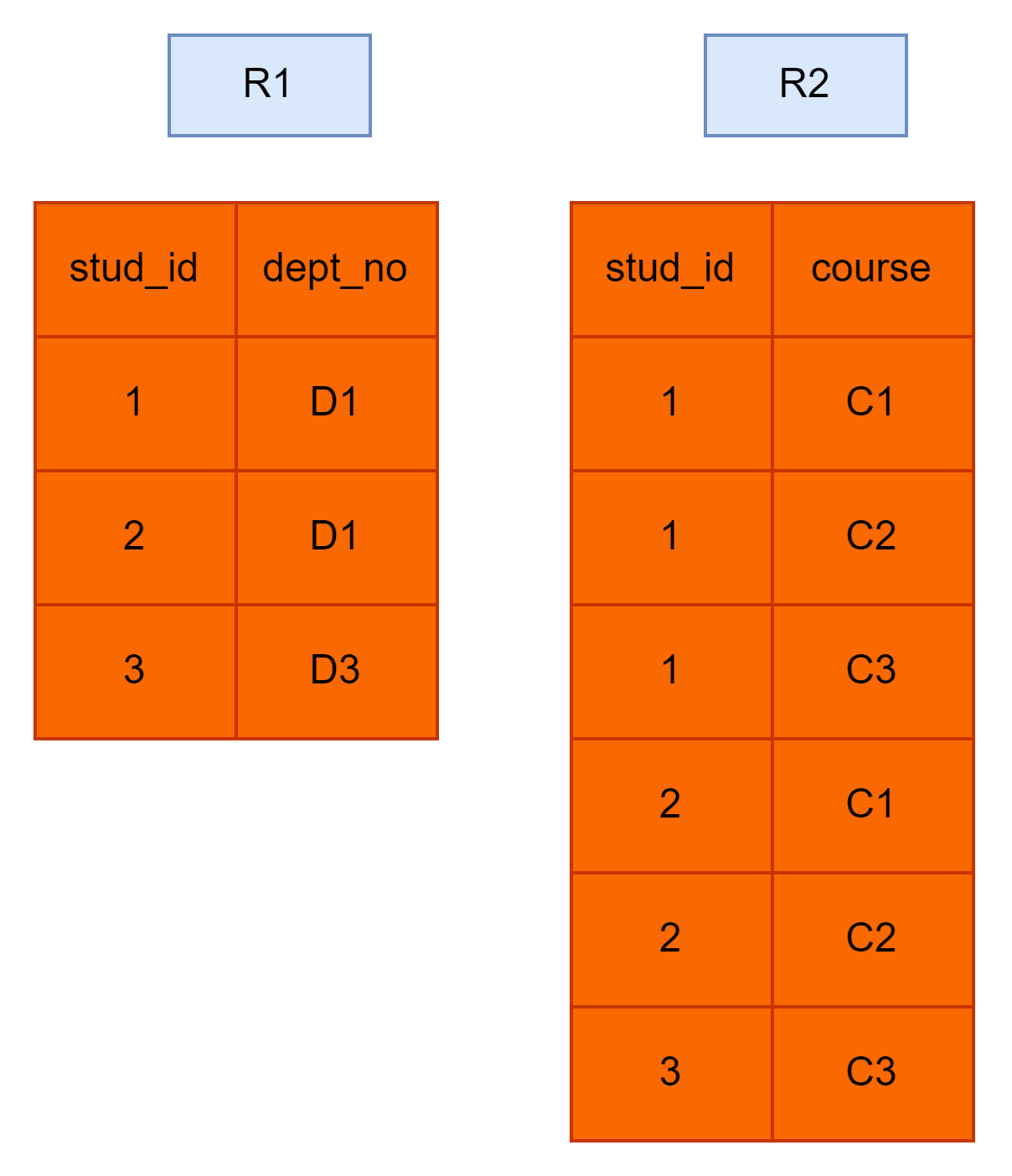# Fourth Normal Form

## Introduction

We have already studied that a relation is in first normal form (1NF) when every attribute has atomic values. A relation is in second normal form (2NF) when there is no partial dependency in the relation. Similarly, the table is in the third normal form (3NF) when there is no transitive dependency in the relation. We also studied that for a table to be in Boyce Codd Normal form (BCNF), for each FD X->Y, X should be a super key.

Most of the redundancies are reduced after the BCNF. However, there can still be some redundancy even after the table is in BCNF. To solve this problem, we have one more normal form known as the Fourth Normal Form (4NF). This article will discuss the fourth normal form. Before we discuss the fourth normal form, it is important to understand the meaning of multivalued dependency.

## Multivalued dependency

We represent multivalued dependency as X->->Y. It means that for a single value of X, multiple values of Y exist. Consider the following students' relation. The attributes are stud_id, dept_no, and the course.From the above table, it can be observed that for a given student (stud_id), there can be more than one course in which the student is enrolled. For every student, there is only one department. We can say that:

1. Stud_id -> dept_no: Each student determines the department in which he/she has taken admission.

2. Stud_id ->-> course: Each student "multi determines" the course. This means that one student can enroll in more than one course.

Formally, multivalued dependency represents a dependency between attributes (say X, Y, and Z) in a relation R, such that for each value of X, there is a set of values of Y and Z. However, Y and Z are independent of each other.

A table must have at least three columns in order to have a multivalued dependency.

Now that we know what multivalued dependencies are, we can see how this can lead to redundancy in the relation and how the fourth normal form can be used to remove this redundancy.

## Fourth Normal FormIn the above table, it can be seen that because of multivalued dependency (stud_id->-> course), the dept_no is repeated every time we have a new tuple. Since a student is in only one department, this data is redundant, i.e., there is unnecessary repetition of the dept_no value, making this database design inefficient.

The fourth normal form (4NF) is used to remove the multivalued dependency. For a relation or table to be in the fourth normal form:

• It must be in Boyce Codd Normal Form (BCNF).
• It should not have any multivalued dependency.

Let us check if the above students' table is in the fourth normal form or not, and if it is not, then how can we convert it into the fourth normal form.

We can observe that the above table is in BCNF. However, there is multivalued dependency. In order to remove this multivalued dependency, we have to "divide" the table into two parts, as shown below.The first table R1 contains stud_id and dept_no as the attributes and the second table R2 has attributes as stud_id and course. Let analyse both the tables.

• R1
• Stud_id is the primary key. The table is in BCNF. There is no multivalued dependency. Hence R1 is in the fourth normal form.
• R2
• The candidate key is (stud_in, course). They both are part of the candidate key. Therefore the table is in BCNF. It can be observed that here a student is enrolled in multiple courses. This gives us the illusion that R2 has a multivalued dependency, which is not true. Firstly in R2, there are only two columns, whereas, for multivalued dependency, at least three columns should be there. Most importantly, there is no attribute that is independent of others. Therefore R2 is also in the fourth normal form

The fourth normal form (4NF) is very useful in reducing redundancy.

## FAQs

1. What is the fourth normal form in DBMS?
It represents the normal form where the table is already in BCNF, and there is no non-trivial multivalued dependency.

2. What is multivalued dependency?
If for a single value of X, there are multiple values of Y, Y is said to be multivalued dependent on X. It is represented as X->->Y.

3. What are the necessary conditions for a table to be in the fourth normal form?
The table should already be in BCNF, and there should be no multivalued dependency.

4. What problem does multivalued dependency cause?
The data becomes redundant.

Take this awesome course from coding ninjas.

## Key takeaways

• There can still be some redundancy even after the table is in BCNF. To solve this problem, we have one more normal form known as the Fourth Normal Form (4NF).
• For a table to be in the fourth normal form, the table should already be in BCNF, and there should be no multivalued dependency.
• We represent multivalued dependency as X->->Y. It means that for a single value of X, multiple values of Y exist.
• Multivalued dependency causes data to be redundant.
• A table must have at least three columns in order to have multivalued dependency.

Never stop learning. Explore the top 100 SQL problems here.

Happy learning!